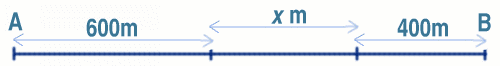#### You may also like### Golden Thoughts

Rectangle PQRS has X and Y on the edges. Triangles PQY, YRX and XSP have equal areas. Prove X and Y divide the sides of PQRS in the golden ratio.### From All Corners

Straight lines are drawn from each corner of a square to the mid points of the opposite sides. Express the area of the octagon that is formed at the centre as a fraction of the area of the square.### Star Gazing

Find the ratio of the outer shaded area to the inner area for a six pointed star and an eight pointed star.

# One and Three

##### Age 14 to 16 Challenge Level:

Two motorboats are travelling up and down a lake at constant speeds and turning at each end without slowing down. They leave opposite ends A and B at the same instant, passing each other, for the first time 600 metres from A and, for the second time, 400 metres from B. How long is the lake (the distance from A to B) and what is the ratio of the speeds of the boats?

We give the solution by Nisha Doshi , Year 9, The Mount School, York. Well done Nisha this is a really good solution.Call the boats $A\prime$ and $B\prime$. The first time they meet, the distances gone are: 600 metres by $A\prime$ and $(x + 400)$ metres by $B\prime$.

The second time the distances are: $(x + 400 + 400)$ metres by $A\prime$ and $(600 + 600 + x)$ metres by $B\prime$.

Since the two speeds are constant, the ratios of the distances travelled each time must be constant, so $$\frac{600}{x+400} = \frac{x+800}{x+1200}$$ which leads to
\begin{eqnarray} 600(x + 1200) &=& (x + 400)(x + 800) \\ 600x + 720000 &=& x^2 + 1200x + 320000 \\ x^2 + 600x - 400000 &=& 0 \\ (x - 400)(x + 1000) &=& 0 \\ \end{eqnarray}
It follows that $x = 400$ metres and that the length of the lake is $600 + 400 + 400 = 1400$ metres.

The ratio of the speeds must be 600 : 800 or 3 : 4.

FOOTNOTE: You can also use the fact that at the first meeting the combined distance travelled is one length of the lake and at the second it is 3 lengths. It follows that if $A\prime$ travels 600 metres by the first meeting it will have travelled three times that distance by the second meeting, that is 1800 metres. This gives $x = 1800 - 600 - 400 - 400 = 400$ and hence the length of the lake is 1400 metres.